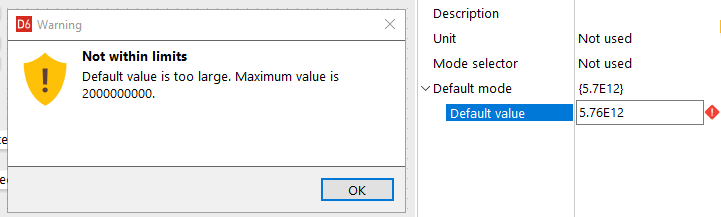0

# Calculation Overflow in Math Channel

I'm trying to use a math channel to do some higher value calculations.  I keep getting a calculation overflow error and it returns a value of 2E09.  I'm assume this is the maximum value for an integer.  But with a real value, the maximum value should be much higher than this.  I would think that I should be getting a real value instead of an integer value.  Is there a way to ensure I'm getting a real value?  Or is something else going on entirely?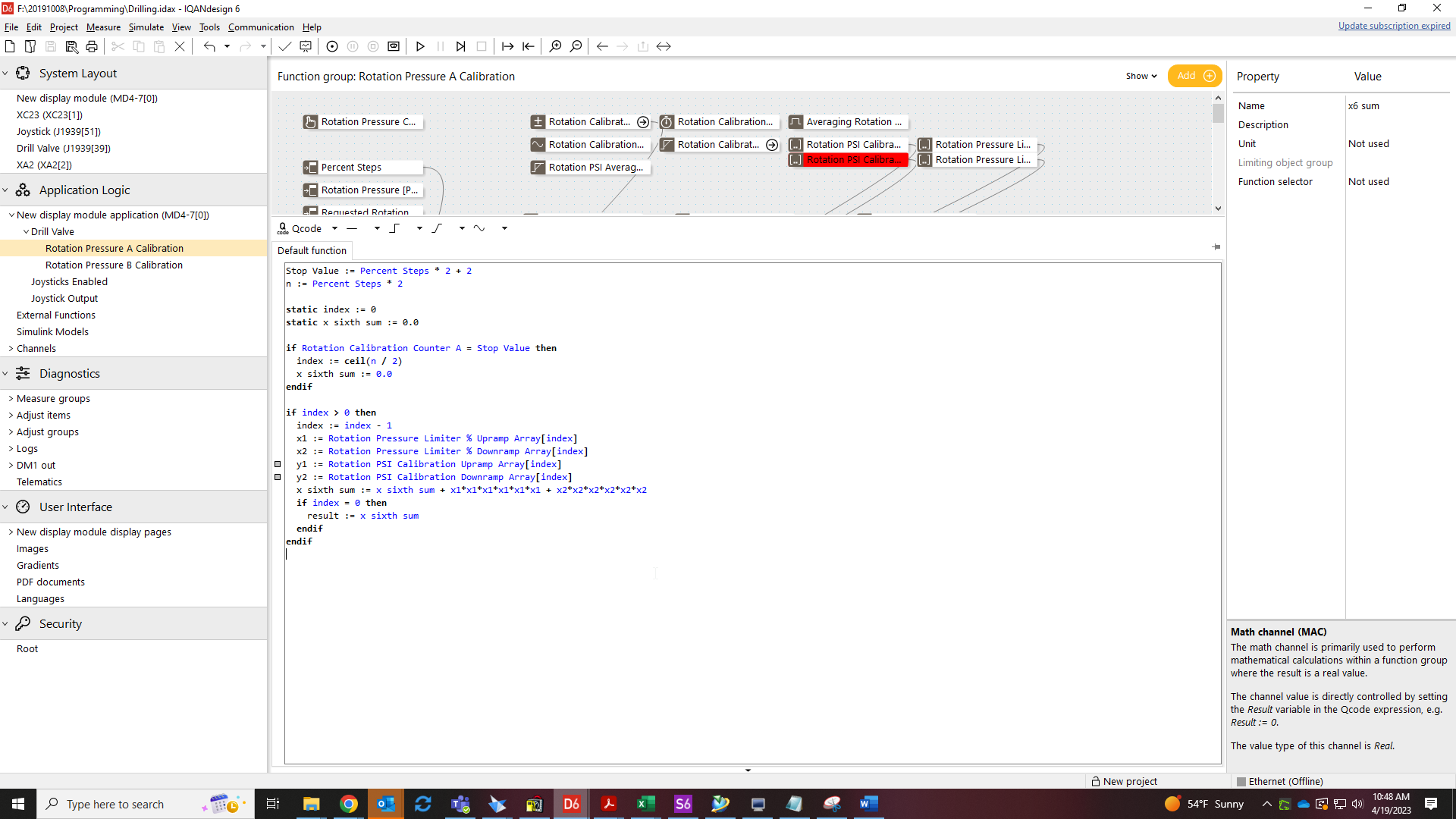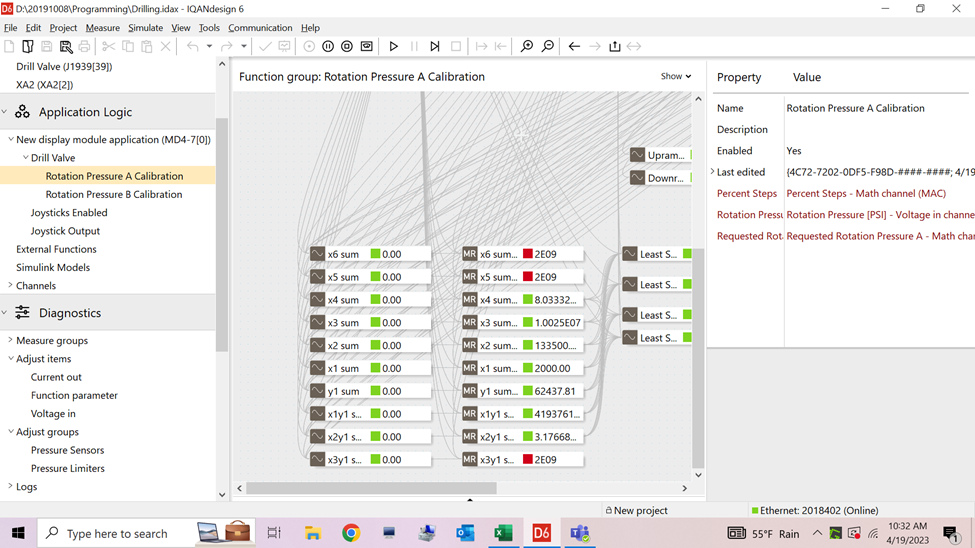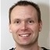You have the value type you intended, 32-bit Real. This can represent values up to 3.4*10^38

Looking at the printscreen from the application, it appears as if you step through indexes of an array and take the values to the power of 6. This can work as long as the values are small, but with too large input values the result will exceed what the 32-bit real can represent.

This is detected as a Calculation overflow.

When a Qcode expression evaluation result in error, the remaining statements in the channel will not be evaluated. The  error status is propagated to the channel, while the channel value remain at the last valid Result.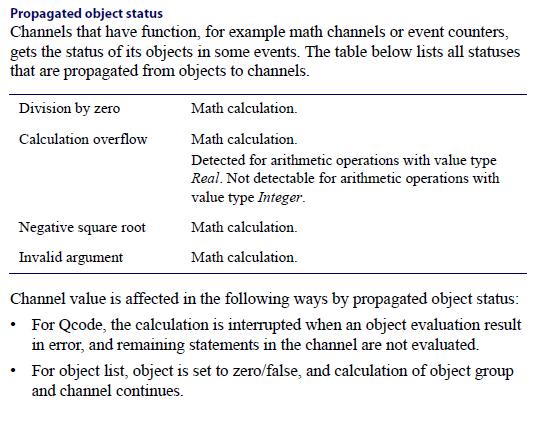Gustav,

The values are smaller than 3.4*10^38. That's why I thought I wasn't getting the correct value type. There are three values that give me calculation overflow and they are all displayed as 2E09. This seems to be more in line with a 32 bit integer and not 32 bit floatingThe values are smaller than 3.4*10^38.  That's why I thought I wasn't getting the correct value type.  There are three values that give me calculation overflow and they are all displayed as 2E09.  This seems to be more in line with a 32 bit integer and not 32 bit floating.From the printscreen that was attached, it looks like there is no limit to how large the variable "x sixth sum" could become, it looks like it increments every cycle that the condition is fulfilled.

A suggestion is to try it in IQANsimulate and step through one cycle at the time. then I think you will see how it quickly grows very large.I know what the value should be.  So I entered it into a function parameter which should be a real value.  It's telling me the value is too large and the maximum is 2000000000.  Why is the maximum this value when the real number value should be 3.4*10^38. If I insert the value into a math channel, it also tells me it's too large.